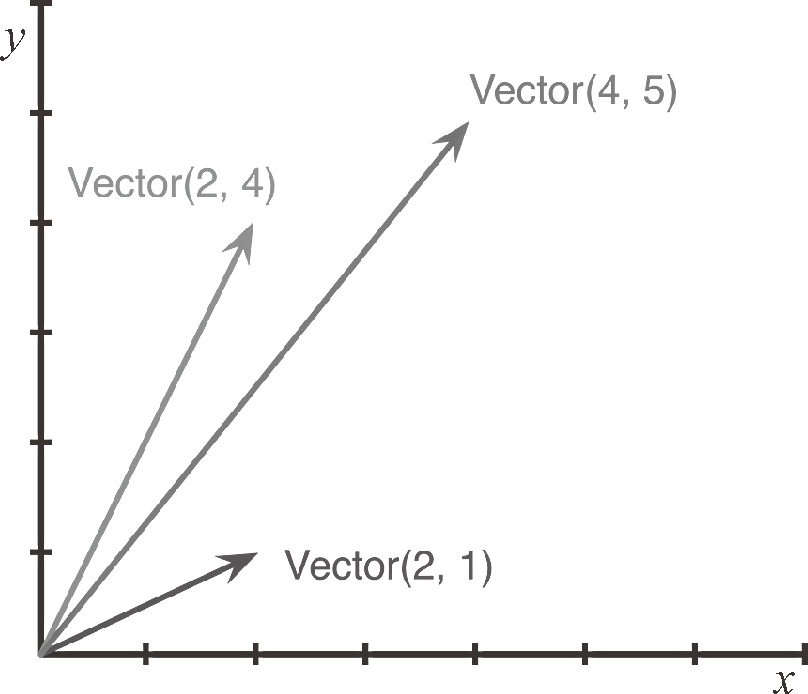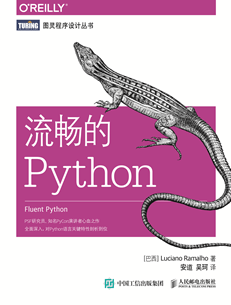# 流畅的 Python(4)：Python 数据模型 1.2.1(模拟数值类型)Python 内置的 complex 类可以用来表示二维向量，但我们这个自定义的类可以扩展到 n 维向量，详见第 14 章。

>>> v1 = Vector(2, 4)
>>> v2 = Vector(2, 1)
>>> v1 + v2
Vector(4, 5)


abs 是一个内置函数，如果输入是整数或者浮点数，它返回的是输入值的绝对值；如果输入是复数（complex number），那么返回这个复数的模。为了保持一致性，我们的 API 在碰到 abs 函数的时候，也应该返回该向量的模：

>>> v = Vector(3, 4)
>>> abs(v)
5.0


1 如果向量与负数相乘，得到的结果向量的方向与原向量相反。——编者注

>>> v * 3
Vector(9, 12)
>>> abs(v * 3)
15.0


from math import hypot

class Vector:

def __init__(self, x=0, y=0):
self.x = x
self.y = y

def __repr__(self):
return 'Vector(%r, %r)' % (self.x, self.y)

def __abs__(self):
return hypot(self.x, self.y)

def __bool__(self):
return bool(abs(self))

x = self.x + other.x
y = self.y + other.y
return Vector(x, y)

def __mul__(self, scalar):
return Vector(self.x * scalar, self.y * scalar)